# Chemical Equations and Reactions Describing Chemical Reactions Chemical

• Slides: 10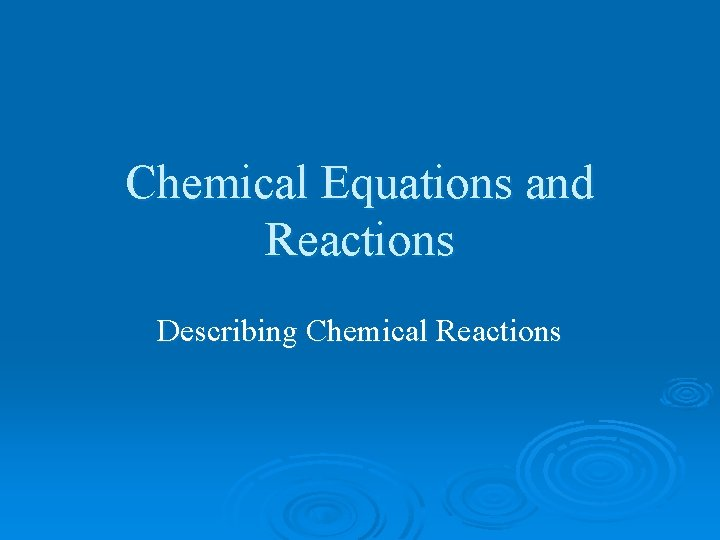Chemical Equations and Reactions Describing Chemical Reactions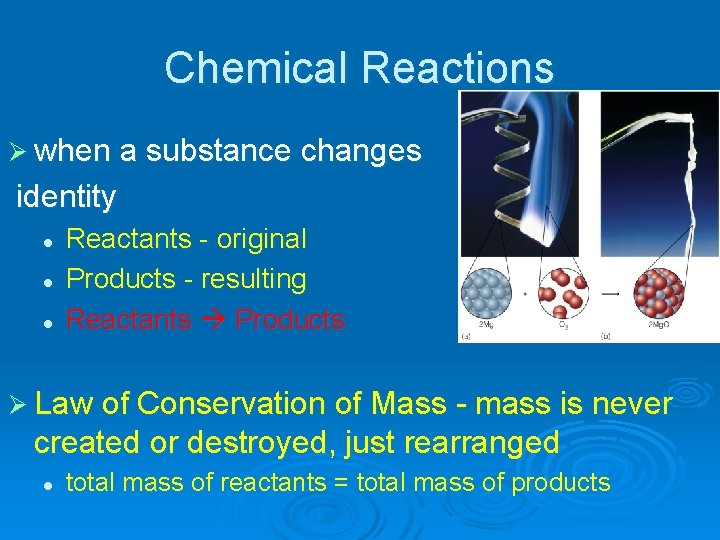Chemical Reactions Ø when a substance changes identity l l l Reactants - original Products - resulting Reactants Products Ø Law of Conservation of Mass - mass is never created or destroyed, just rearranged l total mass of reactants = total mass of productsChemical Reactions Ø chemical equation l l Written form of a chemical reaction uses symbols and formulas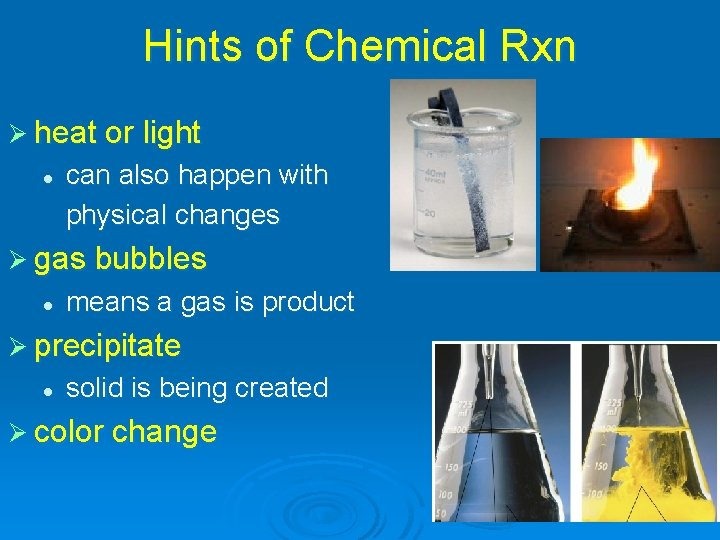Hints of Chemical Rxn Ø heat or light l can also happen with physical changes Ø gas bubbles l means a gas is product Ø precipitate l solid is being created Ø color change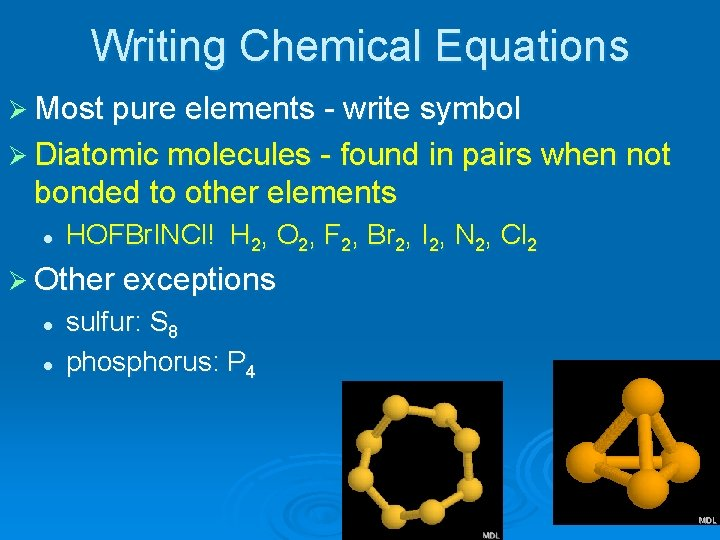Writing Chemical Equations Ø Most pure elements - write symbol Ø Diatomic molecules - found in pairs when not bonded to other elements l HOFBr. INCl! H 2, O 2, F 2, Br 2, I 2, N 2, Cl 2 Ø Other exceptions l l sulfur: S 8 phosphorus: P 4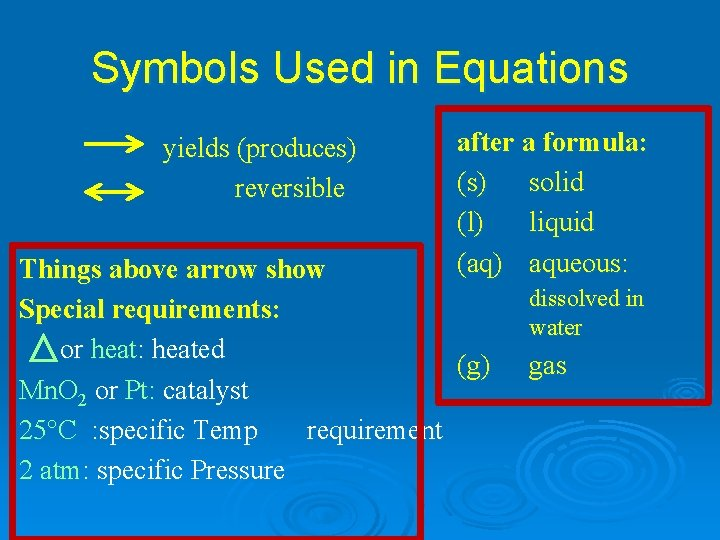Symbols Used in Equations yields (produces) reversible after a formula: (s) solid (l) liquid (aq) aqueous: Things above arrow show Special requirements: or heat: heated (g) Mn. O 2 or Pt: catalyst 25°C : specific Temp requirement 2 atm: specific Pressure dissolved in water gas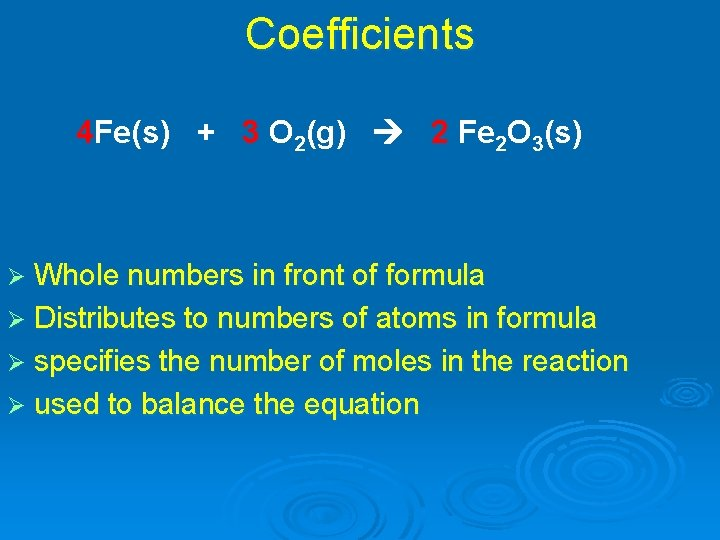Coefficients 4 Fe(s) + 3 O 2(g) 2 Fe 2 O 3(s) Ø Whole numbers in front of formula Ø Distributes to numbers of atoms in formula Ø specifies the number of moles in the reaction Ø used to balance the equation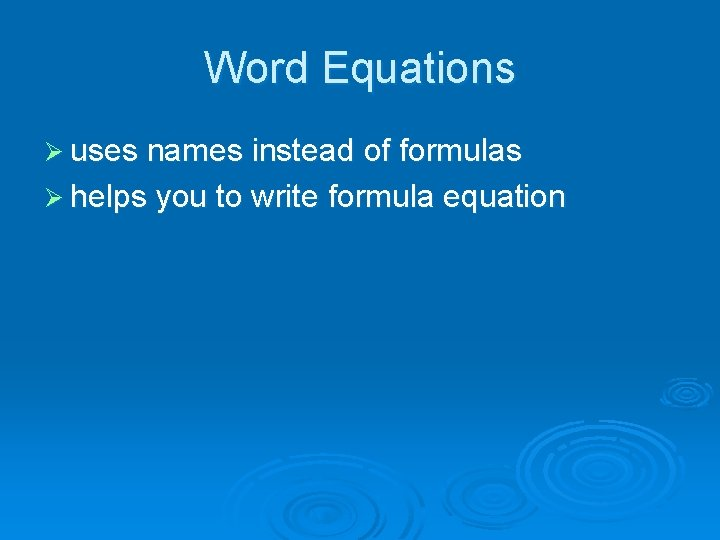Word Equations Ø uses names instead of formulas Ø helps you to write formula equation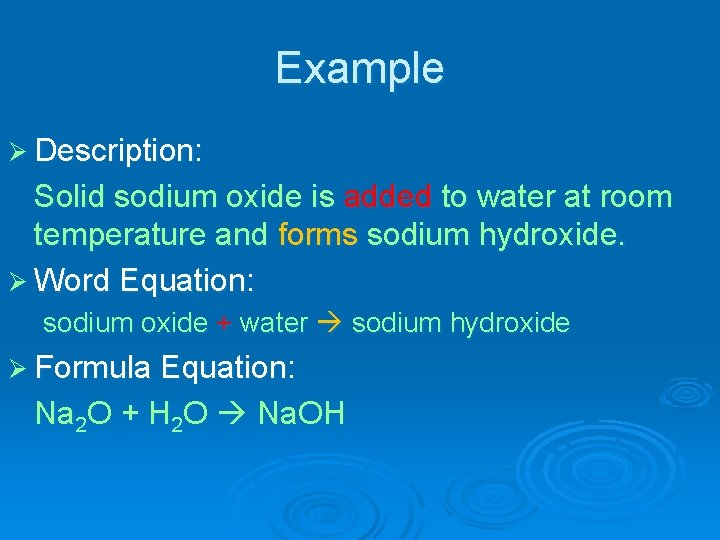Example Ø Description: Solid sodium oxide is added to water at room temperature and forms sodium hydroxide. Ø Word Equation: sodium oxide + water sodium hydroxide Ø Formula Equation: Na 2 O + H 2 O Na. OH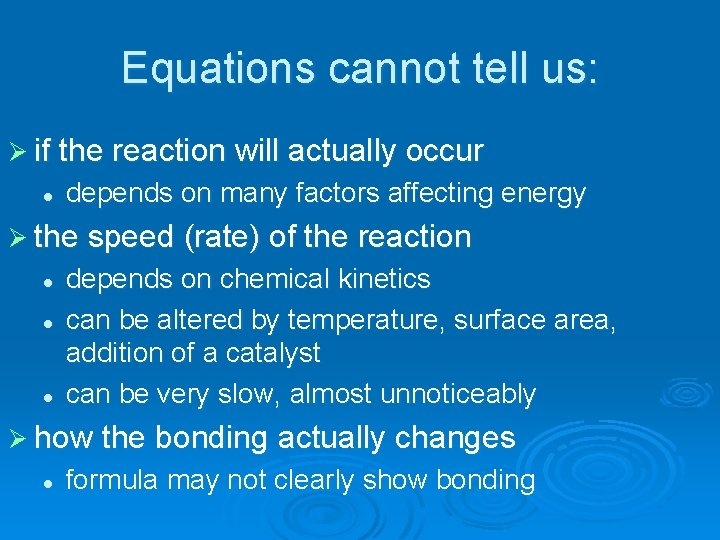Equations cannot tell us: Ø if the reaction will actually occur l depends on many factors affecting energy Ø the speed (rate) of the reaction l l l depends on chemical kinetics can be altered by temperature, surface area, addition of a catalyst can be very slow, almost unnoticeably Ø how the bonding actually changes l formula may not clearly show bonding